GeeksforGeeks App
Open AppBrowser
Continue

## Related Articles

• CBSE Class 11 Chemistry Notes

Chemistry is the study of matter’s transformation from one form to another. These transformations are frequently caused by the interaction of two different types of matter. Certain fundamental rules govern the combination of different elements to form compounds. These rules are known as the law of chemical combination.

When matter reacts or combines with another matter in a chemical reaction, it changes its forms, properties, and characteristics. In chemical reactions, the laws of chemical combination govern the relationship between matter, energy, and transformation. The chemical combinations of elements are governed by five basic laws of chemical combination: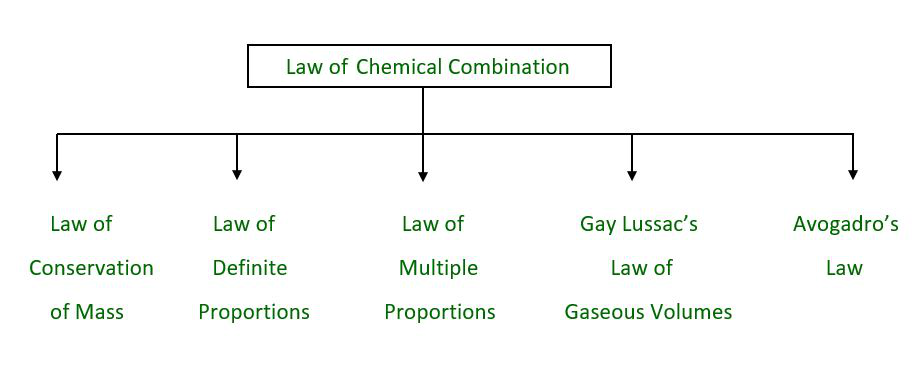### Law of Conservation of Mass

Antoine Lavoisier developed this law in 1789. According to the law, ‘Mass cannot be created or destroyed in a chemical reaction.’

This law states that whenever matter undergoes a chemical or physical change in an enclosed system, the total mass of reacting matter (reactants) is exactly equal to the total mass of reaction products. Because no products or reactants are allowed to exit the system, there is no substance loss and mass is conserved.

Example: Take a look at the formation of a water molecule. A water molecule is formed when hydrogen and oxygen combine.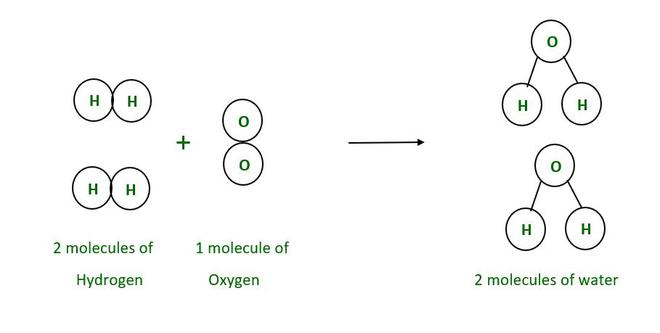In the above example 2 molecules of Hydrogen combines with 1 molecule of Oxygen to form 2 molecules of water.

The masses of H is 1unit and O is 16 unit.

Mass of 2 molecules of Hydrogen (2H2) : 4 unit.

Mass of 1 molecules of Oxygen (O2) : 32 unit.

Mass of 2 molecule of water (2H2O) : 36 unit.

2H    +     O ———>  2H2O

4 unit        32 unit              36 unit

The total mass of the reactants equals the total mass of the products in this case. Furthermore, the number of hydrogen and oxygen atoms in the reactants and products sides is equal.

### Law of Definite Proportions

In 1799, Joseph Louis Proust developed and proved this law. According to the law, ‘the elements are always present in definite proportions by mass in a chemical substance.’

When a compound is broken, the masses of the constituent elements remain in the same proportion, according to the law of constant proportions. Alternatively, in a chemical compound, the elements are always present in definite mass proportions. In simple terms, the percent composition of elements by weight in a given compound will always remain the same regardless of its source, origin, or quantity.

Example: Consider the different molecules of Oxides of Nitrogen.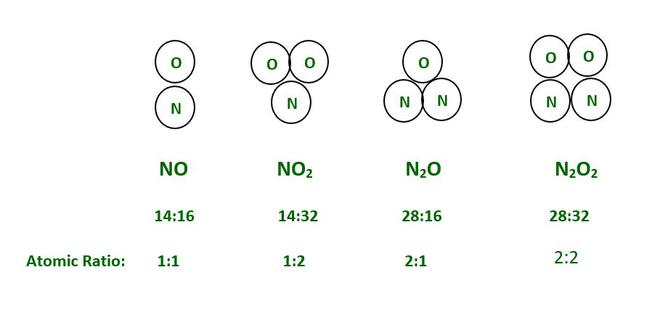In the above example of oxides of Nitrogen if we take any amount of Oxygen and Nitrogen in sample then the ratio of formed NO will always 1:1. Similarly, the ratio for NO2, N2O and N2O2 will be 1:2, 2:1 and 2:2 respectively. This is guaranteed by the law of definite proportions.

### Law of Multiple Proportions

Dalton issued this law in the year 1803. This law states that ‘if two elements combine to form more than one compound, their masses in the reaction are in the ratio of small whole numbers.’

According to the law of multiple proportions, when two elements combine to form more than one compound, the mass of one element combined with a fixed mass of the other element will always be ratios of whole numbers.

Example:

Carbon and oxygen combine to form two distinct compounds (under different circumstances). The first is the most common gas, CO2 (Carbon dioxide), and the second is CO (Carbon monoxide).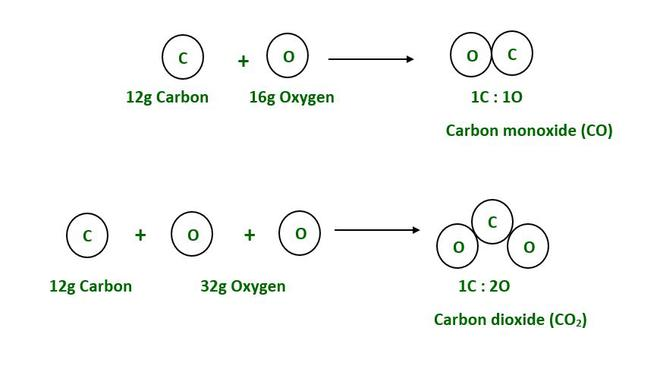We know that,

The mass of Carbon is 12g.

The mass of Oxygen is 16g.

As a result, 12g of carbon combines with 32g of oxygen to form CO2.

Likewise, 12g of carbon reacts with 16g of oxygen to form CO.

As a result, the oxygen ratio in the first and second compounds is 2:1= 32/16 =2, (whole number).

### Gay Lussac’s Law of Gaseous Volumes

Gay Lussac enacted this law based on his observations in 1808. This law states that “when gases are produced or combined in a chemical reaction, they do so in a simple volume ratio provided that all the gases are at the same temperature and pressure.”

This law is regarded as a different type of law of definite proportions. The difference between these two chemical combination laws is that Gay Lussac’s Law is stated in terms of volume, whereas the law of definite proportions is stated in terms of mass.

Example: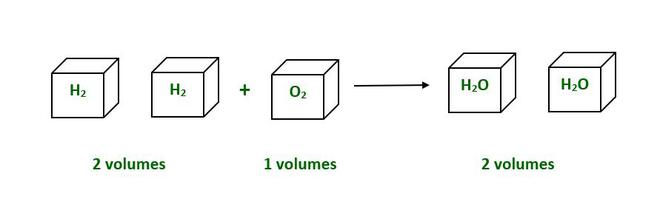In the above example 2 volumes of H2 combines 1 volume of O2 to form 2 volumes of H2O.

H (g)    +     O (g)    ——–>    2H2O  (g)

In 1811, Avogadro proposed this law. According to this law, ‘equal volume of all gases contain the equal number of molecules under the same conditions of temperature and pressure.’ This means that two liters of hydrogen have the same number of molecules as two liters of oxygen at the same temperature and pressure.

Example: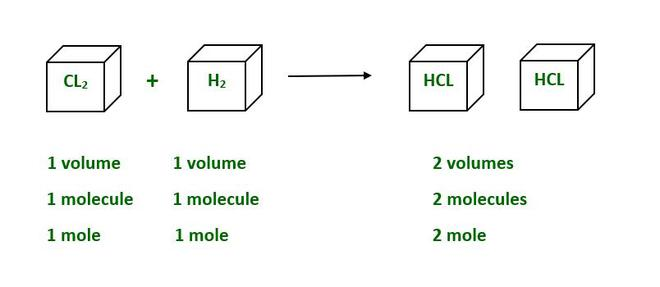Equivalent volumes of different gases contain the same number of molecules at the same temperature and pressure. In the above example CL2 and H₂ has 1 volume each combines to form 2 volume of HCL.

Here,

Mole is a unit of measurement for substance. 1 mole substance contains 6.02214076×10²³ particles.

My Personal Notes arrow_drop_up
Related Tutorials